Question

calculate the percent composition by mass of aluminum hydroxide AL(OH)3 M ynt (in g) of nitrogen...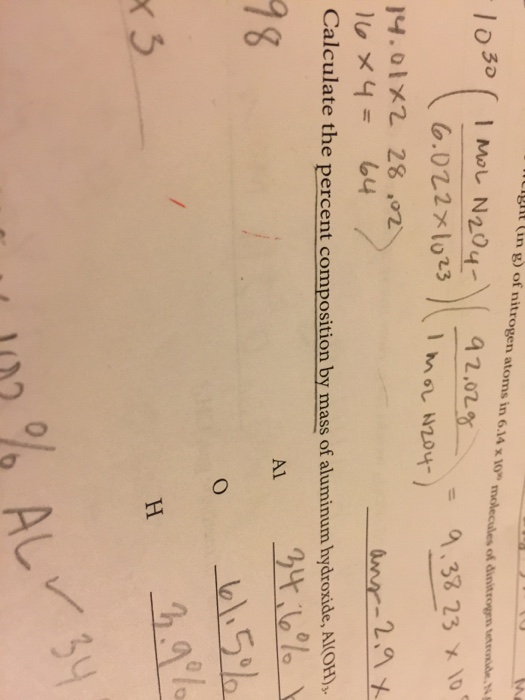calculate the percent composition by mass of aluminum hydroxide AL(OH)3
M ynt (in g) of nitrogen atoms in 6.14 x 10" molecules of dinitrogen anden 9 2.02g Imor 2204-) -1030 ( IMEL N204-) 16.022x1023 = 9.38 23 x 100 14.01X2 28.02 16*4= 64 Calculate the percent composition by mass of aluminum hydroxide, Al(OH) 34:6% ! anr-2,9x Α1 18 61.5% 3.9% x 3 102% ALY

First we will find Molar Mass of Aluminium Hydroxide

Molar Mass of Aluminium Hydroxide Al(OH)3 = (1 x 27) + (3 x 16) + (3 x 1) g/mol

= (27 + 48 + 3) g/mol

= 78 g/mol

Now,

Percentage Composition of Aluminium = (Mass of Aluminium / Molar Mass of Al(OH)3 ) X 100

= (27 / 78) X 100%

= 34.6 %

Percentage Composition of Oxygen = (Mass of Oxygen / Molar Mass of Al(OH)3 ) X 100

= (48 / 78) X 100%

= 61.5 %

Percentage Composition of Hydrogen = (Mass of Hydrogen / Molar Mass of Al(OH)3 ) X 100

= (3 / 78) X 100%

= 3.85 %

Earn Coins

Coins can be redeemed for fabulous gifts.

Similar Homework Help Questions
• 5. Determine the percent composition of oxygen in aluminum sulfate, Al, (SO4)3. The atomic mass of...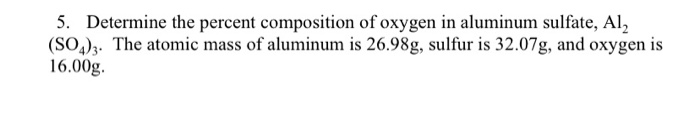5. Determine the percent composition of oxygen in aluminum sulfate, Al, (SO4)3. The atomic mass of aluminum is 26.98g, sulfur is 32.07g, and oxygen is 16.00g.

• 17.(10 pts) Calculate the molar solubility of aluminum hydroxide, Al(OH)3 Ksp = 1.9 x 10”, in...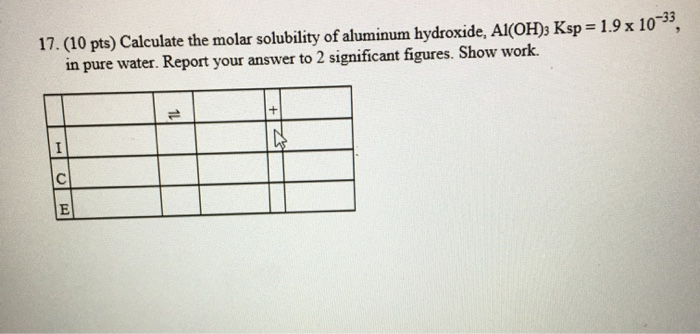17.(10 pts) Calculate the molar solubility of aluminum hydroxide, Al(OH)3 Ksp = 1.9 x 10”, in pure water. Report your answer to 2 significant figures. Show work.

• Calculate the mass of water formed when 0.706 g of aluminum hydroxide reacts with excess hydrochloric...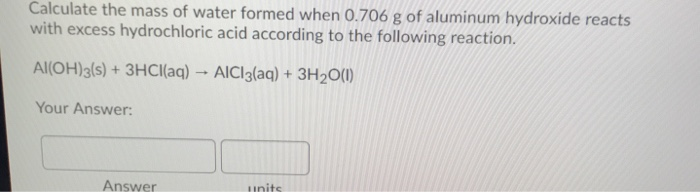Calculate the mass of water formed when 0.706 g of aluminum hydroxide reacts with excess hydrochloric acid according to the following reaction. Al(OH)3(s) + 3HCl(aq) - AlCl3(aq) + 3H2O(1) Your Answer: Answer Hinits

• 11. The solubility product constant for aluminum hydroxide (Al(OH)3) is 3.0 x 10”. Use the information...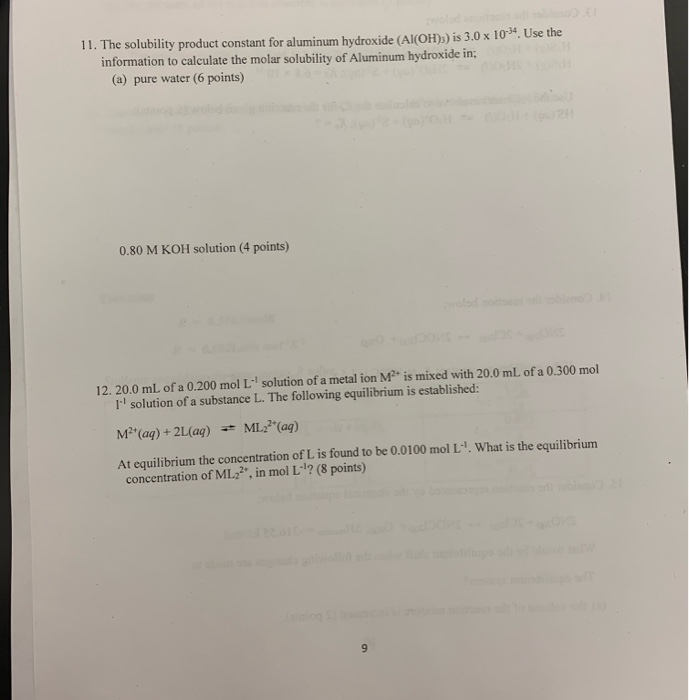11. The solubility product constant for aluminum hydroxide (Al(OH)3) is 3.0 x 10”. Use the information to calculate the molar solubility of Aluminum hydroxide in: (a) pure water (6 points) 0.80 M KOH solution (4 points) 12. 20.0 mL of a 0.200 mol L solution of a metal ion M is mixed with 20.0 mL of a 0.300 mol I'' solution of a substance L. The following equilibrium is established: M (aq) + 2L (aq) + ML(aq) At equilibrium the...